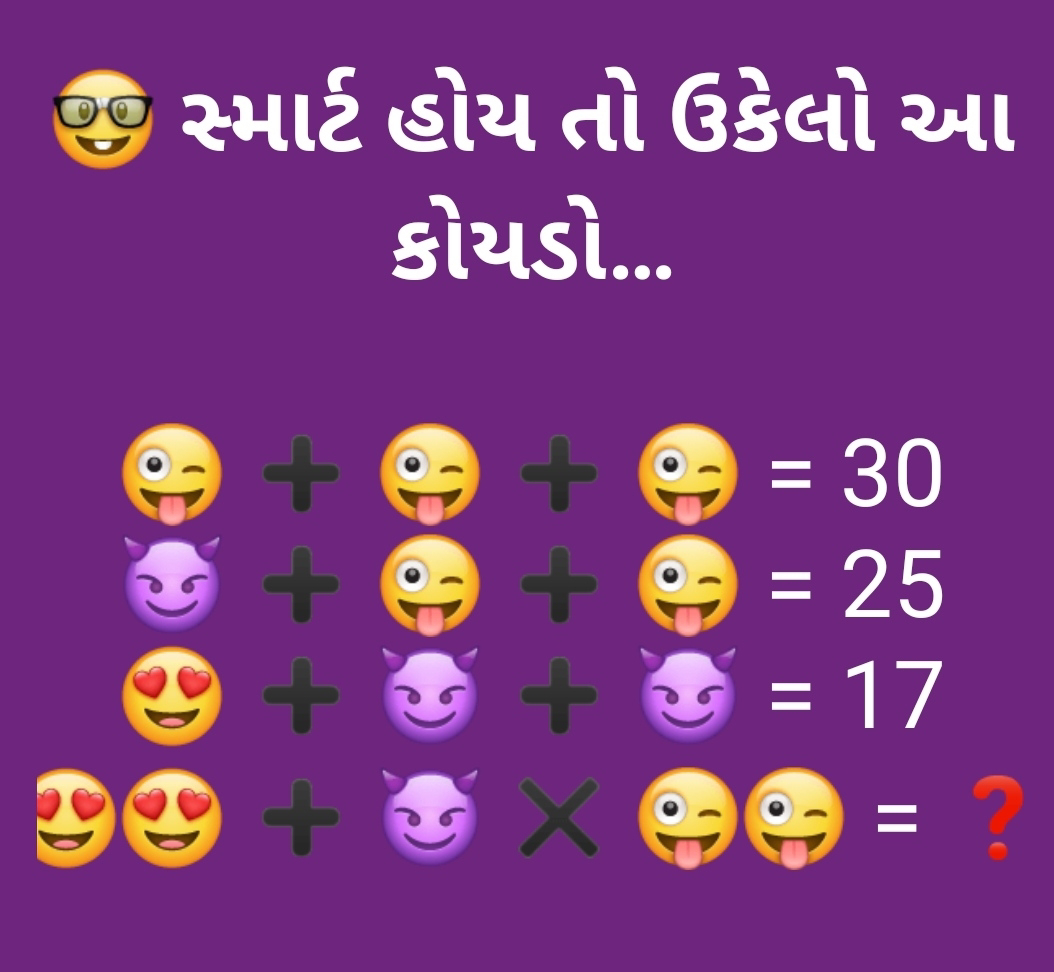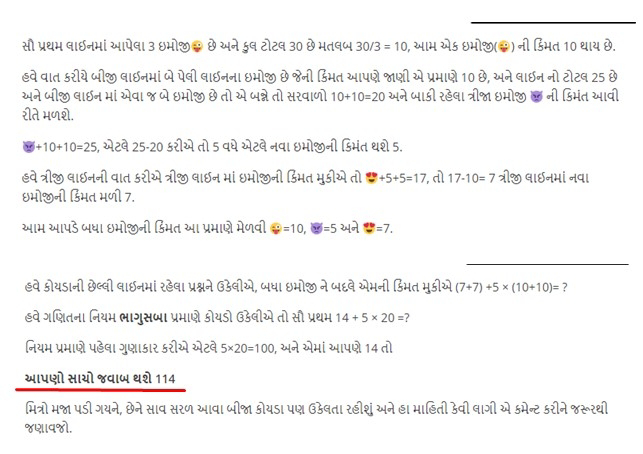Type Here to Get Search Results !

# Maths Amazing Puzzles with Answers

7

## Maths Amazing Puzzles with Answers

puzzle is a game, problem, or toy that tests a person's ingenuity or knowledge. In a puzzle, the solver is expected to put pieces together in a logical way, in order to arrive at the correct or fun solution of the puzzle.There are different genres of puzzles, such as crossword puzzles, word-search puzzles, number puzzles, relational puzzles, and logic puzzles.

Puzzles are often created to be a form of entertainment but they can also arise from serious mathematical or logical problems. In such cases, their solution may be a significant contribution to mathematical research.Etymology

The Oxford English Dictionary dates the word puzzle (as a verb) to the end of the 16th century. Its earliest use documented in the OED was in a book titled The Voyage of Robert Dudley...to the West Indies, 1594–95, narrated by Capt. Wyatt, by himself, and by Abram Kendall, master (published circa 1595). The word later came to be used as a noun, first as an abstract noun meaning 'the state or condition of being puzzled', and later developing the meaning of 'a perplexing problem'. The OED's earliest clear citation in the sense of 'a toy that tests the player's ingenuity' is from Sir Walter Scott's 1814 novel Waverley, referring to a toy known as a "reel in a bottle".

The etymology of the verb puzzle is described by OED as "unknown"; unproven hypotheses regarding its origin include an Old English verb puslian meaning 'pick out', and a derivation of the verb pose.

Tags

1.Very nice sir

2.Last ma be emoji vache symbol mukvani jarur chhe, em kem khabar pde ki be emoji no plus karvano chhe na ki multiply..

Ane jo symbol nathi to simple rite aa rite pan calculate kari sakay.

77 + 5 x 1010 =5127

Jo multiply kariye to

(7x7) +5 x (10x10) = 549

3.10+10+10=30
5+10+10=25
7+5+5=17
14+(5×20)=114

4.5.6.1.1 😜 emoji value is 10 .
Here we have 2 emoji so it's value will be 20.
In the same way 1 😍 value is 7 so, 2 emoji value will be 14.
- Now we will implement multiplication.
5*20=100